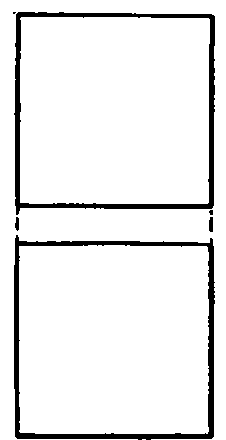## Isometric Of A Cube

In orthographic projection an object has been represented by two or more projections; another system, called isometrical drawing, is often used to show in one view the three dimensions of an object, length (or height), breadth, and thickness. An isometrical drawing of an object, as a cube, is called for brevity the isometric of the cube.

To obtain a view which shows the three dimensions in such a way that measurements may be taken from them, draw the cube in the simple position shown at the left, Fig. 152, with two faces parallel to V; the diagonal from the front upper right-hand corner to the back lower left-hand corner is indicated by the dotted line. Swing the cube around until the diagonal is parallel with V, as shown in the second position. Here the front face is at the right. In the third position the lower end of the diagonal has been raised so that it is parallel to H, becoming thus parallel to both planes. The plan is found by the principles of projection, from the elevation and the preceding plan. The front face is now the lower of the two faces shown in the elevation. From this position the cube is swung around, using the corner as a pivot, until the diagonal is perpendicular to V but still parallel to H. The plan remains the same, except as regards position; while the elevation, obtained by projecting across from the previous elevation, gives the isometrical projection of the cube. The front face is now at the left.

## Distinction Between Isometric Projection And Isometric Drawing

In the last position, as one diagonal is perpendicular to V, it follows that all the faces of the cube make equal angles with V, hence are projected on that plane as equal parallelograms. For the same reason all the edges of the cube are projected in elevation in equal lengths, but, being inclined to V, appear shorter than they actually are on the object. Since they are all equally foreshortened and since a drawing may be made at any scale, it is customary to make all the isometrical lines of a drawing full length. This will give the same proportions, and is much the simpler method. Herein lies the distinction between an isometric projection and an isometric drawing. It will be noticed that the figure may be inscribed in a circle, and that the outline is a perfect hexagon. Hence the lines showing breadth and length are 30-degree lines, while those showing height are vertical.Fig. 152. Development of an Isometric of a Cube.

## True Length Of Lines

Fig. 153 shows the isometric of a cube 1 inch square. All of the edges are shown in their true length, hence all the surfaces appear of the same size. In the figure the edges of the base are inclined at 30 degrees with a T-square line, but this is not always the case. For rectangular objects, such as prisms, cubes, etc., the base edges are at 30 degrees only when the prism or cube is supposed to be in the simplest possible position. The cube in Fig. 153 is supposed to be in the position indicated by plan and elevation in Fig. 154, that is, standing on its base, with two faces parallel to the vertical plane.Fig. 153. Isometric of a Cube.Fig. 154. Plan and Elevation of a Cube.

If the isometric of the cube in the position shown in Fig. 155 were required, it could not be drawn with the base edges at 30 degrees; neither would these edges appear in their true lengths. It follows, then, that in isometrical drawing, true lengths appear only as 30-degree lines or as vertical lines. Edges or lines that in actual projection are either parallel to a T-square line or perpendicular to V, are drawn in isometric as 30-degree lines, full length; and those that are actually vertical are made vertical in isometric, also full length.Fig. 155. Cube of Fig. 154 Rotated 30 Degrees with Vertical Plane.

## Three Isometric Axes

In Fig. 152, lines such as the front vertical edges of the cube and the two base edges are called the three isometric axes. The isometric of objects in oblique positions, as in Fig. 155, can be constructed only by reference to their projections, by methods which will be explained in the section on "Oblique Projection", page 123.

## Applications Of Isometric Projections

In isometric drawing small rectangular objects are more satisfactorily represented than large curved ones. In woodwork, mortises and joints and various parts of framing are well shown in isometric. This system is used also to give a kind of bird's-eye view of mills or factories. It is also used in making sketches of small rectangular pieces of machinery, where it is desirable to give shape and dimensions in one view.